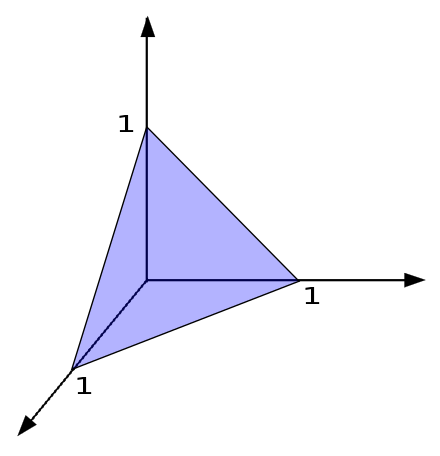# Multiple tetrahedra?

Probability Level 4

$\begin{cases} x+y+z = 1\\ x+y+z = 6\\ x-y-z = 1\\ x-y-z = 6\\ y-z-x = 1\\ y -z-x = 6\\ z-y-x = 1\\ z-y-x = 6\\ \end{cases}$

The above equations define planes in 3 space.

How many ways can you choose four of them so that the enclosed region defines a regular tetrahedron?×